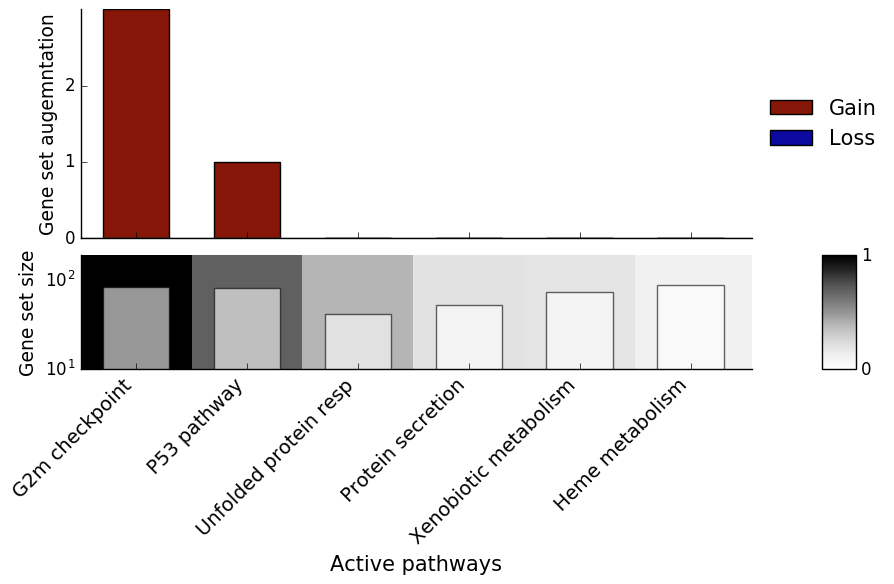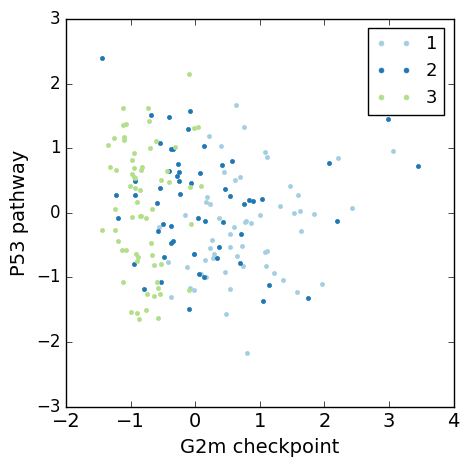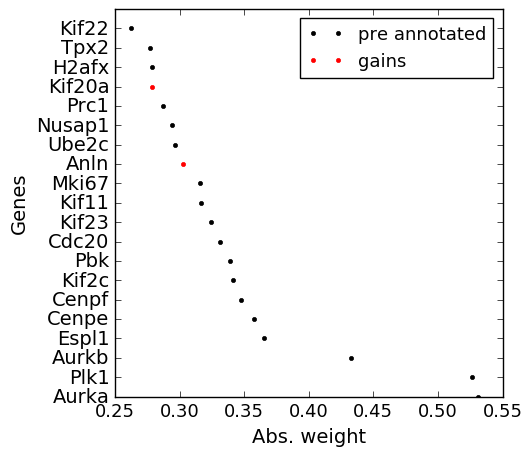slalom tutorial¶

In this notebook we illustrate how slalom can be used to identify biological drivers on the mESC cell cycle staged dataset.

First, we load some modules and set some directories

In :
import os
import slalom
import pdb
%pylab inline

#specify where the hdf5 file is
data_dir = '../data/'
out_dir = './results'
Populating the interactive namespace from numpy and matplotlib

slalom expects an expression file, typically with log transformed gene expression values as well as a gene set annotation. These data can be provided as single hdf5 file, which can be generated using separate R scripts (in the R folder). Alternatively, the expression matrix and the annotation can be loaded as text files in python.

In :
####
#Option 1: load a pre-defined hdf5 file
#We provide an (optional) hdf file with the required data - this was generated using
#the R script write_slalom.R in the R folder
if 0:
annoDB = 'MSigDB'
dFile = os.path.join(data_dir,'Buettneretal2015.hdf5')

####

####
#Option 2: load the annotation and the data directly
if 1:
#Annotation file
annoFile = os.path.join(data_dir,'h.all.v5.0.symbols.gmt.txt') #MSigDB
annoDB   = 'MSigDB'
if not os.path.exists(annoFile):
#Note: the license of MSigDB does not permit redistribution of the raw annotation files.
#hallmark gene sets and place the file in data folder.

#dataFile: csv file with log expresison values
dataFile = os.path.join(data_dir,'Buettneretal.csv.gz') # note that the first column (row names) contains the cell cycle stage in numeric form
####

###alternatively the data can be loaded from the provided hdf5 file
#dFile = 'Buettneretal2015.hdf5'

#print statistics for the loaded dataset
print ("Loaded {:d} cells, {:d} genes".format(data['Y'].shape,data['Y'].shape))
print ("Annotation: {:d} terms".format(len(data['terms'])))
Annotation: 50 terms

Initializing the slalom model¶

In :
#I: indicator matrix that assigns genes to pathways
I = data['I'] #if loaded from the hdf file change to I = data['IMSigDB']
#Y: log expresison values
Y = data['Y']
#terms: ther names of the terms
terms = data['terms']

#gene_ids: the ids of the genes in Y
gene_ids = data['genes']

#initialize FA instance, here using a Gaussian noise model and fitting 3 dense hidden factors
FA = slalom.initFA(Y, terms,I, gene_ids=gene_ids, noise='gauss', nHidden=3, minGenes=15)
/Users/flo/projects/Auto_Bionf/scLVM2/slalom/core.py:392: RuntimeWarning: divide by zero encountered in true_divide
logPi = SP.log(self.Pi[:,m]/(1-self.Pi[:,m]))
/Users/flo/projects/Auto_Bionf/scLVM2/slalom/core.py:394: RuntimeWarning: divide by zero encountered in true_divide
logPi = SP.log(self.Pi[:,m]/(1-self.Pi[:,m]))

Model training¶

In :
#model training
FA.train()

#print diagnostics
FA.printDiagnostics()
iteration 0
iteration 100
iteration 200
iteration 300
iteration 400
iteration 500
iteration 600
iteration 700
Converged after 701 iterations
Maximally  4.6875 % Genes per factor changed.

Next, we plot the results, including factor relevance, gene set augmentation and a scatter plot of the two most relevant factors, in this case G2M Checkpoint and P53 Pathway.

In :
#plot results

fig = plotRelevance(FA)

#get factors; analogous getters are implemented for relevance and weights (see docs)
X = FA.getX(terms=['G2m checkpoint','P53 pathway'])

#scatter plot of the top two factors
fig = plotFactors(X=X, lab=data['lab'], terms=['G2m checkpoint','P53 pathway'], isCont=False)

#the same plot can be generated by passing the FA object to the plot function
#plotFactors(FA=FA,idx1=0,idx2=1, lab = data['lab'], isCont=False )In :
#visualize changes for the G2m checkpoint